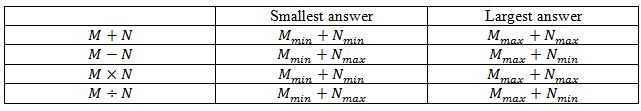# Upper and lower bound

### Upper and lower bound

#### Lessons• Introduction
Introduction to upper and lower bounds
a)
How to find the upper and lower bounds?

b)
What is "Limits of accuracy"?

• 1.
Evaluating the Upper and Lower Bounds
Find the upper and lower bounds for each of the following scenarios:
a)
The time it takes Dennis to finish a 1km-race is 130s, rounded to the nearest 10 seconds.

b)
The size of a watch face measures 4cm to the nearest centimeter.

c)
Thomas weighs 72.6kg, accurate to 1 decimal place.

d)
The maximum speed of Kevin's car is 200mph, rounded to 1 significant figure.

• 2.
Understanding the Limits of Accuracy
In Sylvia's garden, the average width of a willow tree trunk is 136cm while that of a maple tree trunk is 172cm, both numbers corrected to the nearest centimeter. Find the greatest possible difference in their widths.

• 3.
A rectangle with length 7cm and width 3cm is given. If both sides are measured to the nearest cm, find the smallest possible area.

• 4.
Michael takes 43s, to the nearest second, to finish a 400m sprint, measured to the nearest 10m. What is his fastest possible average speed?